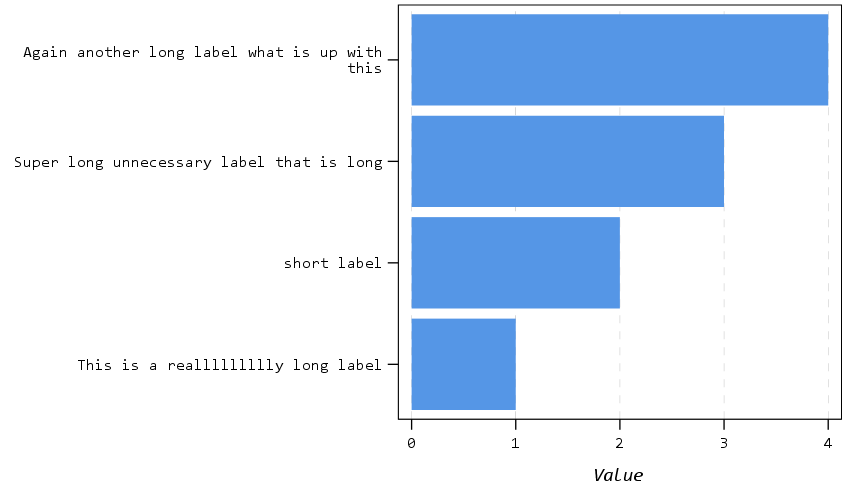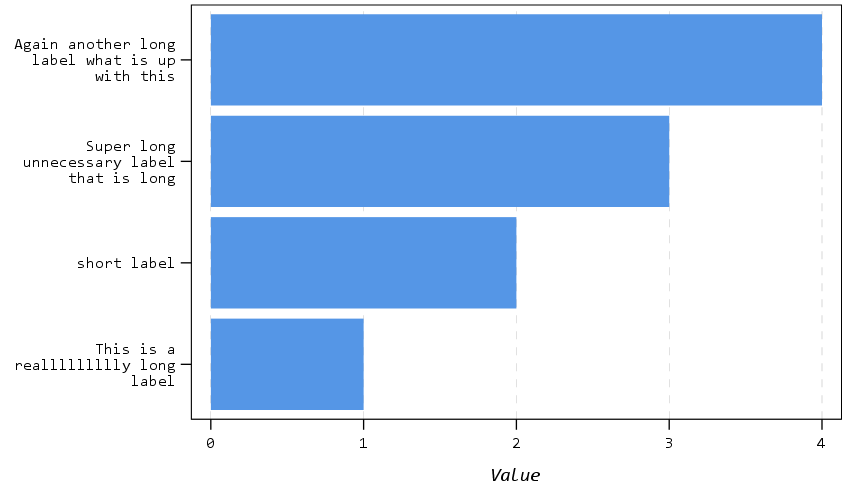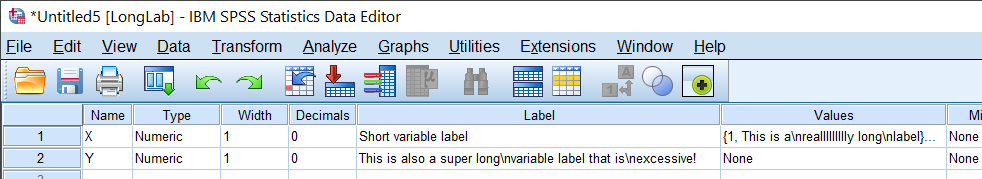# Python f string number formatting and SPSS break long labels

Another quick blog post, as moving is not 100% crazy all the time now, but I need a vacation after all that work. So two things in this blog post: formatting numeric f strings in python, and breaking long labels in SPSS meta-data.

## Python f-string numeric formatting

This is super simple, but I can never remember it (so making a quick blog post for my own reference). As of python 3.6, you can use f-strings to do simple text substitution. So if you do:

``````x = 2/3
sub_str = f'This proportion is {x}'
print(sub_str)``````

Then we will get printed out `This proportion is 0.6666666666666666`. So packing global items inside of `{}` expands within the f string. While for more serious string subsitution (like creating parameterized SQL queries), I like to use string templates, these f-strings are very nice to print short messages to the console or make annotations in graphs.

Part of this note is that I never remember how to format these strings. If you are working with integers it is not a big deal, but as you can see above I often do not want to print out all those decimals inside my particular message. A simple way to format the strings are:

``f'This proportion is {x:.2f}'``

And this prints out to two decimal places `'This proportion is 0.67'`. If you have very big numbers (say revenue), you can do something like:

``f'This value is \${x*10000:,.0f}'``

Which prints out `'This value is \$6,667'` (so you can modify objects in place, to say change a proportion to a percentage).

Note also to folks that you can have multi-line f-strings by using triple quotes, e.g.:

``````f'''This is a super
long f-string for {x:.2f}
on multiple lines!'''``````

But one annoying this is that you need to keep the whitespace correct inside of functions even inside the triple string. So those are cases I like using string templates. But another option is to break up the string and use line breaks via `\n`.

``````long_str = (f'This is line 1\n'
f'Proportion is {x:.1f}\n'
f'This is line 3')
print(long_str)``````

Which prints out:

``````This is line 1
Proportion is 0.7
This is line 3``````

You could do the line breaks however, either at the beginning of each line or at the end of each line.

## SPSS break long labels

This was in reference to a project where I was working with survey data, and for various graphs I needed to break up long labels. So here is an example to illustrate the problem.

``````* Creating simple data to illustrate.
DATA LIST FREE / X Y(2F1.0).
BEGIN DATA
1 1
2 2
3 3
4 4
END DATA.
DATASET NAME LongLab.
VALUE LABELS X
1 'This is a reallllllllly long label'
2 'short label'
3 'Super long unnecessary label that is long'
4 'Again another long label what is up with this'
.
VARIABLE LABELS
X 'Short variable label'
Y 'This is also a super long variable label that is excessive!'
.
EXECUTE.

GGRAPH
/GRAPHDATASET NAME="g" VARIABLES=X Y
/GRAPHSPEC SOURCE=INLINE.
BEGIN GPL
SOURCE: s=userSource(id("g"))
DATA: X=col(source(s), name("X"), unit.category())
DATA: Y=col(source(s), name("Y"))
COORD: rect(dim(1,2), transpose())
GUIDE: axis(dim(1))
GUIDE: axis(dim(2), label("Value"))
SCALE: linear(dim(2), include(0))
ELEMENT: interval(position(X*Y))
END GPL.``````So you can see, SPSS shrinks the data to accommodate the long labels. (I don’t know how to control the behavior in the graph or the chart template itself, so not sure why only `this` gets wrapped for the first label.) So we can use the `\n` line break trick again in SPSS to get these to split where we prefer. Here are some python functions to do the splitting (which I am sure can be improved upon), as well as to apply the splits to the current SPSS dataset. You can decide the split where you want the line to be broken, and so if a word goes above that split level it wraps to the next line.

``````* Now some python to wrap long labels.
BEGIN PROGRAM PYTHON3.
import spss, spssaux

# Splits a long string with line breaks
def long_str(x,split):
split_str = x.split(" ")
cum = len(split_str)
cum_str = split_str
for s in split_str[1:]:
cum += len(s) + 1
if cum <= split:
cum_str += " " + s
else:
cum_str += r"\n" + s
cum = len(s)
return cum_str

# This grabs all of the variables in the current SPSS dataset
varList = [spss.GetVariableName(i) for i in range(spss.GetVariableCount())]

# This looks at the VALUE LABELS and splits them up on multiple lines
def split_vallab(vList, lsplit):
vardict = spssaux.VariableDict()
for v in vardict:
if v in vList:
vls= v.ValueLabels.keys()
if vls:
for k in vls:
ss = long_str(v.ValueLabels[k], lsplit)
if ss != v.ValueLabels[k]:
vn = v.VariableName
cmd = '''ADD VALUE LABELS %(vn)s %(k)s \'%(ss)s\'.''' % ( locals() )
spss.Submit(cmd)

# I run this to split up the value labels
split_vallab(varList, 20)

# This function is for VARIABLE LABELS
def split_varlab(vList,lsplit):
for i,v in enumerate(vList):
vlab = spss.GetVariableLabel(i)
if len(vlab) > 0:
slab = long_str(vlab, lsplit)
if slab != vlab:
cmd = '''VARIABLE LABELS %(v)s \'%(slab)s\'.''' % ( locals() )
spss.Submit(cmd)

# I don't run this right now, as I don't need it
split_varlab(varList, 30)
END PROGRAM.``````

And now we can re-run our same graph command, and it is alittle nicer:

``````GGRAPH
/GRAPHDATASET NAME="g" VARIABLES=X Y
/GRAPHSPEC SOURCE=INLINE.
BEGIN GPL
SOURCE: s=userSource(id("g"))
DATA: X=col(source(s), name("X"), unit.category())
DATA: Y=col(source(s), name("Y"))
COORD: rect(dim(1,2), transpose())
GUIDE: axis(dim(1))
GUIDE: axis(dim(2), label("Value"))
SCALE: linear(dim(2), include(0))
ELEMENT: interval(position(X*Y))
END GPL.``````And you can also go to the variable view to see my inserted line breaks:SPSS still does some auto-intelligence when to wrap lines in tables/graphs (so if you do `DISPLAY DICTIONARY.` it will still wrap the X variable label in my default tables, even though I have no line break). But this gives you at least a slight bit of more control over charts/tables.